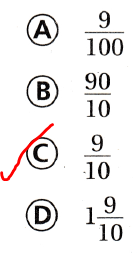# Texas Go Math Grade 4 Lesson 2.3 Answer Key Relate Tenths and Decimals

Refer to our Texas Go Math Grade 4 Answer Key Pdf to score good marks in the exams. Test yourself by practicing the problems from Texas Go Math Grade 4 Lesson 2.3 Answer Key Relate Tenths and Decimals.

## Texas Go Math Grade 4 Lesson 2.3 Answer Key Relate Tenths and Decimals

Essential Question

How can you record tenths as fractions and decimals?
We know that,
The place-value position of tenths present after the first place of the decimal point
Hence,
The representation of the tenths in the form of a decimal is: 0.1
The representation of the tenths in the form of a fraction is: $$\frac{1}{10}$$

Unlock the problem

Ty is reading a book about metamorphic rocks. He has read $$\frac{7}{10}$$ of the book. What decimal describes the part of the book Ty has read?

A decimal is a number with one or more digits to the right of the decimal point. You can write tenths and hundredths as fractions or decimals.

Math Talk

Mathematical Processes:
Explain how the size of one whole is related to the size of one-tenth?
Divide 1 whole into 10 equal parts. or 1 tenth. In the decimal form, each part has a value equal to 0.1 (read as zero point one)

One Way Use a model and a place-value chart.

Fraction
Shade $$\frac{7}{10}$$ of the model.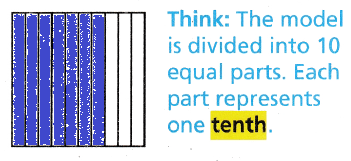Write: 0.7

Decimal
$$\frac{7}{10}$$ is 7 tenths.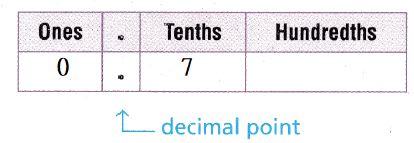Write: 0.7

Another Way: Use a number line.

Label the number line with decimals that are equivalent to the fractions. Locate the point $$\frac{7}{10}$$.Now,
From the above Number line,
0.7 names the same amount as $$\frac{7}{10}$$.
So,
Ty read 0.7 of the book.

How can you write 0.1 as a fraction? Explain.
The given decimal number is: 0.1
Now,
We know that,
When we have 1 digit after the decimal number, the representation of that decimal number into a fraction is: $$\frac{Decimal number without points}{10}$$
Hence, from the above,
We can conclude that
The representation of 0.1 as a fraction is: $$\frac{1}{10}$$

Tara rode her bicycle 1$$\frac{6}{10}$$ miles. What decimal describes how far she rode her bicycle?

You have already written a fraction as a decimal. You can also write a mixed number as a decimal.

Another Way: Use a number line.

Label the number line with equivalent mixed numbers and decimals. Locate the point 1$$\frac{6}{10}$$.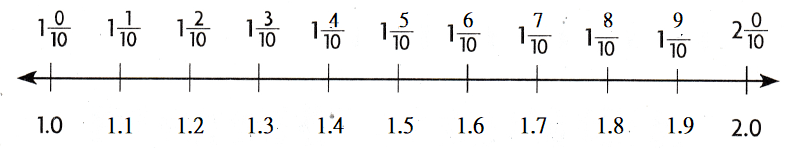Now,
From the above Number line,
1$$\frac{0}{10}$$ names the same amount as 1
So,
Tara rode her bicycle 1.6 miles.

Share and Show

Question 1.
Write five tenths as a fraction and as a decimal.
Fraction: _________ Decimal: _________The given models are:Now,
Now,
The representation of five tenths in the given models is: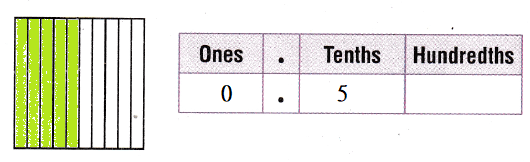Now,
From the above models,
We can observe that
The representation of five tenths in the form of a fraction is: (The number of shaded parts) ÷ (The number of total parts)
So,
The representation of five tenths in the form of a fraction is: $$\frac{5}{10}$$
Hence, from the above,
We can conclude that
The representation of five tenths in the form of a fraction is: $$\frac{5}{10}$$
The representation of five tenths in the form of a decimal is: 0.5

Write the fraction or mixed number and the decimal shown by the model.

Question 2.The given model is:Now,
We know that,
For the representation of a model,
We will consider a “Flat” i.e., whole rectangle as 1
We will consider a “Long” i.e., a part of the whole rectangle as 0.1
Now,
From the above model,
We can observe that
The representation of a model into a fraction = (The number of shaded parts) ÷(The total number of parts)
So,
The representation of the given model into a fraction = $$\frac{10}{10}$$ + $$\frac{10}{10}$$ + $$\frac{2}{10}$$
= $$\frac{10 + 10 + 2}{10}$$
= $$\frac{22}{10}$$
So,
The representation of the given model into a decimal number = 1 + 1 + (2 × 0.1)
= 2.2
Hence, from the above,
We can conclude that
The representation of the given model into a fraction is: $$\frac{22}{10}$$
The representation of the given model into a decimal number is: 2.2

Question 3.The given model is: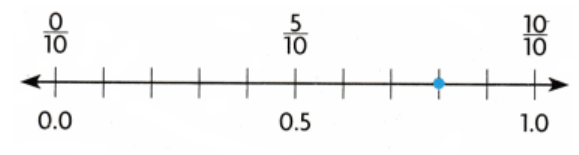Now,
The representation of the complete model is:Now,
From the above model,
We can observe that
The representation of the marked point in the form of a fraction is: $$\frac{8}{10}$$
The representation of the marked point in the form of a decimal number is: 0.8
Hence, from the above,
We can conclude that
The representation of the marked point in the form of a fraction is: $$\frac{8}{10}$$
The representation of the marked point in the form of a decimal number is: 0.8

Math Talk

Mathematical Processes
How can you write 1$$\frac{3}{10}$$ decimal? Explain.
The given mixed number is: 1$$\frac{3}{10}$$
Now,
We know that,
The representation of a mixed number in the form of a decimal number = Whole number + Fraction
Now,
1$$\frac{3}{10}$$ = 1 + $$\frac{3}{10}$$
= 1 + 0.3
= 1.3
Hence, from the above,
We can conclude that
The representation of 1$$\frac{3}{10}$$ as a decimal number is: 1.3

Problem Solving

Use the table for 4-7.Question 4.
What part of the rocks listed ¡n the table are igneous? Write your answer as a decimal.
The given table is:Now,
From the above table,
We can observe that
The part of the rocks that are Igneous = (The number of Igneous rocks) ÷ (The total number of rocks)
= $$\frac{5}{10}$$ part of the rocks
Now,
The representation of the part of the rocks that are Igneous in the decimal form is: 0.5
Hence, from the above,
We can conclude that
The representation of the part of the rocks that are Igneous in the decimal form is: 0.5

Question 5.
Sedimentary rocks make up what part of Ramon’s collection? Write your answer as a fraction and in word form.
The given table is:Now,
From the above table,
We can observe that
The part of the Sedimentary rocks in Ramon’s collection = (The number of Sedimentary rocks) ÷ (The total number of rocks)
= $$\frac{3}{10}$$
So,
The representation of the part of the Sedimentary rocks in Ramon’s collection in the form of a fraction is: $$\frac{3}{10}$$
The representation of the part of the Sedimentary rocks in Ramon’s collection in the Word form is: Three tenths
Hence, from the above,
We can conclude that
The representation of the part of the Sedimentary rocks in Ramon’s collection in the form of a fraction is: $$\frac{3}{10}$$
The representation of the part of the Sedimentary rocks in Ramon’s collection in the Word form is: Three tenths

Question 6.
H.O.T. Representations
What part of the rocks listed in the table is metamorphic? Write your answer as a fraction and as a decimal.
The given table is:Now,
From the above table,
We can observe that
The part of the Metamorphic rocks that are listed in the given table = (The number of Metamorphic rocks) ÷ (The total number of rocks)
= $$\frac{2}{10}$$
So,
The representation of the part of the Mtamorphic rocks that are listed in the given table in the form of a fraction is: $$\frac{2}{10}$$
The representation of the part of the Mtamorphic rocks that are listed in the given table in the form of a decimal number is: 0.2
Hence, from the above,
We can conclude that
The representation of the part of the Mtamorphic rocks that are listed in the given table in the form of a fraction is: $$\frac{2}{10}$$
The representation of the part of the Mtamorphic rocks that are listed in the given table in the form of a decimal number is: 0.2

Question 7.
Multi-Step What’s the Error?
Niki wrote the following sentence in her report: “Metamorphic rocks make up 2.0 of Ramon’s rock collection.” Describe her error and give the correct answer.
The given table is:Now,
From the above table,
We can observe that
The Metamorphic rocks made up of 2 parts of the total Ramon’s collection
So,
The representation of the part of the Metamorphic rocks in the form of a fraction is: $$\frac{2}{10}$$
But,
According to Niki,
Metamorphic rocks make up 2.0 of Ramon’s rock collection
So,
The error is:
Niki did not written the above sentence in a proper way
Metamorphic rocks make up 2.0 parts of Ramon’s rock collection
Hence, from the above,
We can conclude that
The error made by Niki is:
Niki did not written the above sentence in a proper way
Metamorphic rocks make up 2.0 parts of Ramon’s rock collection

Question 8.
Which decimal is the same as $$\frac{1}{10}$$? $$\frac{6}{10}$$? $$\frac{3}{10}$$?
The given decimal numbers are:
$$\frac{1}{10}$$, $$\frac{6}{10}$$, and $$\frac{3}{10}$$
So,
The representations of the given fractions in the form of decimal numbers respectively is: 0.1, 0.6, and 0.3
Hence, from the above,
We can conclude that
$$\frac{1}{10}$$ is the same as: 0.1
$$\frac{6}{10}$$ is the same as: 0.6
$$\frac{3}{10}$$ is the same as: 0.3

Question 9.
Josh wrote $$\frac{3}{10}$$ of his paper on Thursday night and $$\frac{5}{10}$$ of his paper on Friday night. How much of his paper did he complete on Friday night, written as a decimal?
It is given that
Josh wrote $$\frac{3}{10}$$ of his paper on Thursday night and $$\frac{5}{10}$$ of his paper on Friday night
Now,
According to the given information,
The amount of paper did Josh completed on Friday night = $$\frac{3}{10}$$ + $$\frac{5}{10}$$
= $$\frac{3 + 5}{10}$$
= $$\frac{8}{10}$$
= 0.8
Hence, from the above,
We can conclude that
The amount of paper did Josh completed on Friday night in the form of a decimal number is: 0.8

Question 10.
Narwhal whales can weigh as much as 1$$\frac{8}{10}$$ tons. What is 1$$\frac{8}{10}$$ written as a decimal?
(A) 8.10
(B) 10.1
(C) 1.10
(D) 1.8
It is given that
Narwhal whales can weigh as much as 1$$\frac{8}{10}$$ tons
Now,
The given mixed number is: 1$$\frac{8}{10}$$
Now,
1$$\frac{8}{10}$$ = 1 + $$\frac{8}{10}$$
= 1 + 0.8
= 1.8
Hence, from the above,
We can conclude that
The representation of 1$$\frac{8}{10}$$ as a decimal is: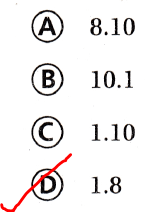Question 11.
Multi-Step What fraction and decimal are shown by the point?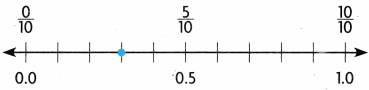(A) $$\frac{3}{10}$$ and 0.3
(B) [ latex]\frac{3}{100}[/latex] and 0.03
(C) $$\frac{8}{10}$$ and 0.08
(D) $$\frac{2}{10}$$ and 0.2
The given model is:Now,
The representation of the complete model is: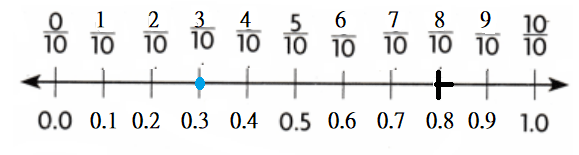Hence, from the above,
We can conclude that
The fraction and decimal that are shown by the give point is: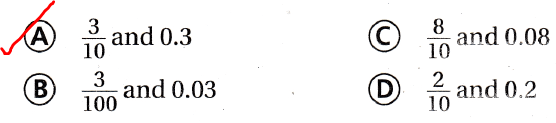Question 12.
Which shows a way to write 0.7 as a fraction?
(A) $$\frac{7}{100}$$
(B) $$\frac{2}{10}$$
(C) $$\frac{7}{10}$$
(D) 1 $$\frac{7}{10}$$
The given decimal number is: 0.7
So,
The representation of 0.7 in the form of a fraction is: $$\frac{7}{10}$$
Hence, from the above,
We can conclude that
A way to write 0.7 as a fraction is: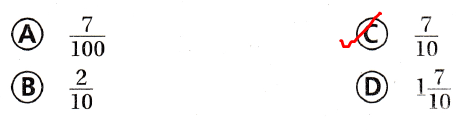Texas Test Prep

Question 13.
Rosa has a bookshelf where she stores her book collection. Four-tenths of her books are mystery books. What is this amount written as a decimal?
(A) 4.0
(B) 0.4
(C) 40.0
(D) 0.04
It is given that
Rosa has a bookshelf where she stores her book collection. Four-tenths of her books are mystery books
Now,
According to the given representation,
The representation of four-tenths in the form of a decimal number is: 0.4
Hence, from the above,
We can conclude that
The amount of Rosa’s books hat are mystery books in the form of a decimal number is:### Texas Go Math Grade 4 Lesson 2.3 Homework and Practice Answer Key

Write the fraction or mixed number as a decimal

Question 1.
$$\frac{6}{10}$$
The given fraction is: $$\frac{6}{10}$$
Now,
According to the given information,
The representation of the given fraction in the form of a decimal is: 0.6
Hence, from the above,
We can conclude that
The representation of the given fraction in the form of a decimal is: 0.6

Question 2.
$$\frac{1}{10}$$
The given fraction is: $$\frac{1}{10}$$
Now,
According to the given information,
The representation of the given fraction in the form of a decimal is: 0.1
Hence, from the above,
We can conclude that
The representation of the given fraction in the form of a decimal is: 0.1

Question 3.
3$$\frac{9}{10}$$
The given mixed number is: 3$$\frac{9}{10}$$
Now,
According to the given information,
3$$\frac{9}{10}$$ = 3 + $$\frac{9}{10}$$
= 3 + 0.9
= 3.9
So,
The representation of the given mixed number in the form of a decimal is: 3.9
Hence, from the above,
We can conclude that
The representation of the given number in the form of a decimal is: 3.9

Problem Solving

Bradley has 10 coins in his pocket. Use the picture of the coins for 4-7.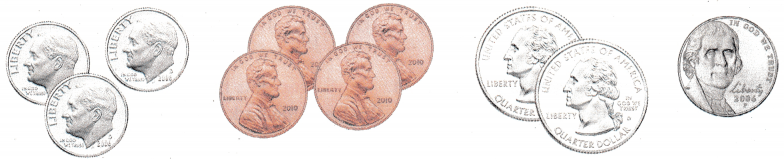Question 4.
What part of the coins are dimes? Write your answer as a decimal.
It is given that
Bradley has 10 coins in his pocket
Now,
The given model is:Now,
From the above model,
We can observe that
The part of the coins that are dimes = (The number of dimes) ÷(The total number of coins)
= $$\frac{4}{10}$$
= 0.4
Hence, from the above,
We can conclude that
The part of the coins that are dimes in the decimal form is: 0.4

Question 5.
Quarters make tip what part of the group of coins? Write your answer as a fraction and in word form.
It is given that
Bradley has 10 coins in his pocket
Now,
The given model is:Now,
From the above model,
We can observe that
The part of the coins that are Quarters = (The number of Quarters) ÷(The total number of coins)
= $$\frac{2}{10}$$
So,
The part of the coins that are Quarters in the Word form is: Two tenths
Hence, from the above,
We can conclude that
The part of the coins that are Quarters in the form of a fraction is: $$\frac{2}{10}$$
The part of the coins that are Quarters in the Word form is: Two tenths

Question 6.
What part of the coins shown are pennies? Write your answer as a fraction and as a decimal.
It is given that
Bradley has 10 coins in his pocket
Now,
The given model is:Now,
From the above model,
We can observe that
The part of the coins that are pennies = (The number of pennies) ÷(The total number of coins)
= $$\frac{3}{10}$$
= 0.3
Hence, from the above,
We can conclude that
The part of the coins that are pennies in the decimal form is: 0.3
The part of the coins that are pennies in the form of a fraction is: $$\frac{3}{10}$$

Question 7.
Amy said that nickels make up $$\frac{1}{9}$$ of Bradley’s coins. What error did Amy make?
It is given that
Amy said that nickels make up $$\frac{1}{9}$$ of Bradley’s coins
Now,
The given model is:Now,
From the above model,
We can observe that
The part of the coins that are nickels = (The number of nickels) ÷(The total number of coins)
= $$\frac{4}{10}$$
= 0.4
But,
According to Amy,
Nickels are made up of $$\frac{1}{9}$$ of Bradley’s coins
But,
There are total 10 coins
So,
The error is:
Amy did not count the total number of coins correctly
Hence, from the above,
We can conclude that
The error made by Amy is:
Amy did not count the total number of coins correctly

Lesson Check

Question 8.
Kristen rode her bicycle 2$$\frac{7}{10}$$ kilometers to school. What is the decimal for 2$$\frac{7}{10}$$ ?
(A) 2.10
(B) 27.1
(C) 2.7
(D) 7.210
It is given that
Kristen rode her bicycle 2$$\frac{7}{10}$$ kilometers to school
Now,
The given mixed number is: 2$$\frac{7}{10}$$
Now,
According to the given information,
2$$\frac{7}{10}$$ = 2 + $$\frac{7}{10}$$
= 2 + 0.7
= 2.7
Hence, from the above,
We can conclude that
The decimal form of 2$$\frac{7}{10}$$ is: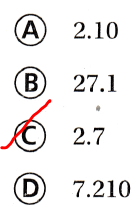Question 9.
Multi-Step
A flat represents 1. Which names the mixed number and the decimal shown by the model?(A) 2$$\frac{9}{10}$$, 2.9
(B) 2$$\frac{9}{100}$$, 2.09
(C) $$\frac{29}{100}$$, 0.29
(D) 1$$\frac{9}{10}$$, 1.9
It is given that
A “Flat” represents 1
Now,
The given model is:Now,
From the above model,
We can observe that
The representation of the fraction for the given model = (The number of shaded parts) ÷ (The total number of parts)
= $$\frac{29}{10}$$
= 2$$\frac{9}{10}$$
= 2 + $$\frac{9}{10}$$
= 2 + 0.9
= 2.9
Hence, from the above,
We can conclude that
The representation of the mixed number and the decimal number for the given model is: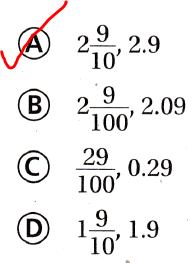Question 10.
Which shows eight-tenths written as a fraction and as a decimal?
(A) $$\frac{8}{10}$$, 0.08
(B) $$\frac{8}{100}$$, 8
(C) $$\frac{8}{100}$$, 0.8
(D) $$\frac{8}{10}$$, 0.8
The given Word form is:
Eight tenths
Now,
According to the given information,
The representation of the given Word form in the form of a decimal number is: 0.8
The representation of the given Word form in the form of a fraction is: $$\frac{8}{10}$$
Hence, from the above,
We can conclude that
The representation of eight-tenths in the form of a fraction and a decimal number is: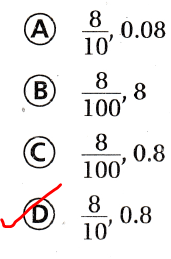Question 11.
Multi-Step
It is 1 kilometer from Owen’s house to the park. The number line shows the distance he has walked. What is the decimal for the distance he has left to walk?(A) 0.8
(B) 0.2
(C) 2
(D) 8
It is given that
It is 1 kilometer from Owen’s house to the park. The number line shows the distance he has walked.
Now,
The given model is:Now,
According to the given information,
The distance that Owen has to walk = (The total distance Owen has to walk) – (The distance Owen has walked)
= 1 – 0.8
= 0.2
Hence, from the above,
We can conclude that
The representation of the distance Owen has to walk in the form of a decimal number is: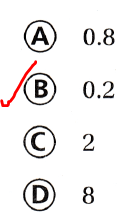Question 12.
Which shows a way to write 0.9 as a fraction?
(A) $$\frac{9}{100}$$
(B) $$\frac{90}{10}$$
(C) $$\frac{9}{10}$$
(D) 1$$\frac{9}{10}$$
The representation of 0.9 in the form of a fraction is: $$\frac{9}{10}$$